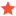Teach Target On SurfaceMichaelw46Junior MemberPosts: 9 Threads: 6 Joined: Feb 2019 Reputation: 1 05-07-2019, 07:45 PM In the RoboDK GUI application under the 'Program' drop-down menu there is an option called 'Teach Target(s) on Surface' which automatically orients the robot tool perpendicular to the surface of an object and gives the user the option to save a target on the surface of that object. I am trying to write a Python code that does the same thing given coordinates of a point on the surface of the object rather than having the user select the target manually, but there does not appear to be any implementation of the 'Teach Target(s) on Surface' option in the Python API. Is there any way to access this option through the API? And if not, how could something similar be implemented using the API? Find ReplyAlbertAdministratorPosts: 398 Threads: 0 Joined: Apr 2018 Reputation: 10 05-09-2019, 11:56 PM Yes, this is possible. You can extract the selected point point of an object as an XYZijk (6D array as XYZ position and ijk normal vector of the surface at that point).  This is possible using SelectedFeature and GetPoints. More information here: https://robodk.com/doc/en/PythonAPI/robo...tedFeature You can iteratively check if the user selected a specific object: Code:```from robolink import *    # API to communicate with RoboDK # Start RoboDK API RDK = Robolink() OBJECT = RDK.ItemUserPick("Select an object", ITEM_TYPE_OBJECT) while True:    pause(0.2)    is_selected, feature_type, feature_id = OBJECT.SelectedFeature()    if not is_selected:        print("Nothing Selected")        continue        point_mouse, name_feature = OBJECT.GetPoints(FEATURE_SURFACE)    print("Selected %i (%i): %s  %s" % (feature_id, feature_type, str(point_mouse), name_feature))``` GetPoints returns a 6D vector as xyzijk. You can then assume the normal of the surface is the Z axis and create a pose using a function like this one: https://robodk.com/doc/en/PythonAPI/robo...xis_2_pose This is a more complete example in C#: Code:```// get the robot reference frame RoboDK.Item robot_ref = ROBOT.getLink(ITEM_TYPE_FRAME); if (!robot_ref.Valid()) {    Console.WriteLine("The robot doesn't have a reference frame selected. Selecting a robot reference frame (or make a reference frame active is required to add a target).");    return; } int feature_type = -1; int feature_id = -1; // Remember the information relating to the selected point (XYZ and surface normal). // These values are retrieved in Absolute coordinates (with respect to the station). double[] point_xyz = null; double[] point_ijk = null; while (true) {    var obj_selected = RDK.GetSelectedItems();    if (obj_selected.Count == 1 && obj_selected.Type() == ITEM_TYPE_OBJECT)    {        var obj = obj_selected;        // RDK.SetSelectedItems(); // ideally we need this function to clear the selection        var is_Selected = obj.SelectedFeature(out feature_type, out feature_id);        if (is_Selected && feature_type == RoboDK.FEATURE_SURFACE)        {            Mat point_list;            string description = obj.GetPoints(feature_type, feature_id, out point_list);            // great, we got the point from the surface. This will be size 6x1            Console.WriteLine("Point information: " + description);            if (point_list.cols < 1 || point_list.rows < 6)            {                // something is wrong! This should not happen....                Console.WriteLine(point_list.ToString());                continue;            }            double[] value = point_list.GetCol(0).ToDoubles();            point_xyz = new double[] { value, value, value };            // invert the IJK values (RoboDK provides the normal coming out of the surface but we usually want the Z axis to go into the object)            point_ijk = new double[] { -value, -value, -value };            Mat obj_pose_abs = obj.PoseAbs();            // Calculate the point in Absolute coordinates (with respect to the station)            point_xyz = obj_pose_abs * point_xyz;            point_ijk = obj_pose_abs.Rot3x3() * point_ijk;            break;        }    } } // Calculate the absolute pose of the robot reference Mat ref_pose_abs = robot_ref.PoseAbs(); // Calculate the absolute pose of the robot tool Mat robot_pose_abs = ref_pose_abs * robot_ref.Pose(); // Calculate the robot pose for the selected target and use the tool Y axis as a reference // (we try to get the pose that has the Y axis as close as possible as the current robot position) Mat pose_surface_abs = Mat.xyzijk_2_pose(point_xyz, point_ijk, robot_pose_abs.VY()); if (!pose_surface_abs.IsHomogeneous()) {    Console.WriteLine("Something went wrong");    return; } // calculate the pose of the target (relative to the reference frame) Mat pose_surface_rel = ref_pose_abs.inv() * pose_surface_abs; // add a target and update the pose var target_new = RDK.AddTarget("T1", robot_ref, ROBOT); target_new.setAsCartesianTarget(); target_new.setJoints(ROBOT.Joints()); // this is only important if we want to remember the current configuration target_new.setPose(pose_surface_rel);``` Find Reply